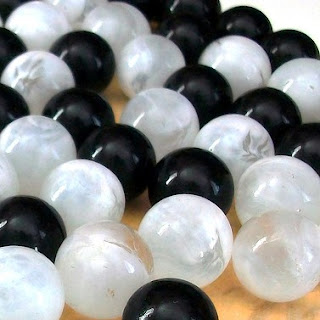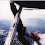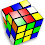## Saturday, June 16, 2012

### GeekDad Puzzle of the Week: When Are the Odds Even?GeekDad Puzzle of the Week: When Are the Odds Even?:
Q: If we have a bag containing equal numbers of black and white marbles, and we pull out one marble, the odds of it being black are even. If we have a bag containing 120 marbles, 85 of which are black, the odds of us pulling out two marbles and them both being black is also even — (85/120)x(84/119) = 0.5 or 50%.

If the largest bag we have can hold 1,000,000 marbles, for how many sets of marbles (i.e., the 120 marbles described above are one set) can we pull two marbles and have a 50% chance of them being the same designated color? Are there any sets of marbles for which we can pull three marbles and have a 50% chance of them being the same designated color? If so, how many?
After the solution is revealed, I'll post the details of my answer.

In the first case you are essentially looking for integer solutions to:
a(a-1) = 2b(b-1)

There are EIGHT sets under 1 million that will result in even odds when 2 balls are drawn.

4 marbles (3 black) --> 4 x 3 = 2(3 x 2)
21 marbles (15 black) --> 21 x 20 = 2(15 x 14)
120 marbles (85 black) --> 120 x 119 = 2(85 x 84)
697 marbles (493 black) --> 697 x 696 = 2(493 x 492)
4,060 marbles (2,871 black) --> 4,060 x 4,059 = 2(2,871 x 2,870)
23,661 marbles (16,731 black) --> 23,661 x 23,660 = 2(16,731 x 16,730)
137,904 marbles (97,513 black) --> 137,904 x 137,903 = 2(97,513 x 97,512)
803,761 marbles (568,345 black) --> 803,761 x 803,760 = 2(568,345 x 568,344)

Interestingly, the next number in each sequence can be computed as follows:
a(n) = 6a(n-1) - a(n-2) - 2

So for example, the next numbers in the sequence would be:
Total balls: 6 x 803,761 - 137,904 - 2 = 4,684,660 marbles
Black balls: 6 x 568,345 - 97,513 - 2 = 3,312,555 black

Integer sequences: A011900 and A046090

In the second case you are looking for integer solutions to:
a(a-1)(a-2) = 2b(b-1)(b-2)

There is only ONE set under 1 million that will result in even odds when 3 balls are drawn.

6 marbles (5 black) --> 6 x 5 x 4 = 2(5 x 4 x 3)

1.Blaine!

2.Answer? LOL! I can handle math, but have no idea what the conditions of this puzzle really are. Is the 1,000,000 total to be made up of many sets of 120 marbles each? Are we free to put any number of marbles in a "set"? How many colors are to be used? I think I'll just wait until the answer is posted to find out what the question really is.

1.The puzzle is asking you to think of a set of marbles in a bag... say 3 marbles. Can you make the proportion of black and white marbles such that the chance of drawing 2 black marbles is exactly 50%? If you had 2 black marbles and 1 white marble, the probability of drawing 2 black marbles would be 2/3 x 1/2 = 1/3. So that's not one of the possible sets. So basically it is asking for all the possible amounts of marbles (from 3 marbles up to 1 million marbles) is it possible to proportion the marbles so that the chance of drawing two black marbles is exactly 50%? Is there only one set (85 black marbles out of a total of 120) or are there more? And if so, what are those sets? Finally, if you figure that out, can you answer the puzzle for drawing *3* black marbles from the bags? The answer will be revealed soon, and I'll add my own thoughts.

3.I sensed right away that this puzzle involves one of my favorite series, the half-companion Pell numbers over the Pell numbers, i.e. the rational approximations to the square root of 2:

(*SIGH* How I wish I could use <font face="Courier">)

1 1 3 7 17 41 99 239 577 1393 3363
- - - - -- -- -- --- --- ---- ----
0 1 2 5 12 29 70 169 408 985 2378

(I started with the illegal fraction 1/0 only because it gives 1st case of
numerator^2 = 2*denominator^2 +/- 1)

Of course I was right. This series DOES INDEED play into the answer to this puzzle.

4.I was gonna post a comment showing how the Pell numbers and half-companion Pell numbers are involved in the answer, but since I can neither employ

<FONT face="Courier">, nor use <TABLE> tags,
I therefore just post the following URL:
http://users.az.com/~jwaters/Puzzles/GeekDad_2012-06-17.htm, which will direct anyone interested to an HTML document which DOES use those tools to show the correlation. Enjoy.

For NPR puzzle posts, don't post the answer or any hints that could lead to the answer before the deadline (usually Thursday at 3pm ET). If you know the answer, submit it to NPR, but don't give it away here.

You may provide indirect hints to the answer to show you know it, but make sure they don't assist with solving. You can openly discuss your hints and the answer after the deadline. Thank you.